Create a new printableGeometry
Math Worksheets

Sample - Click above to make a new math worksheet (PDF).
 Name _____________________________Date ___________________
Angle Relationships
Find the measure of the angle.

 1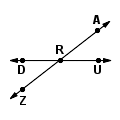m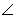URA = _________mDRZ = 382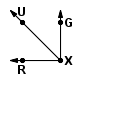mGXU = _________mUXR = 453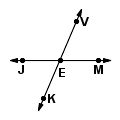mJEK = _________mJEV = 1134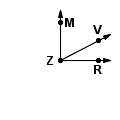mMZV = _________mVZR = 275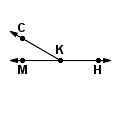mMKC = _________mHKC = 1506 * This is a pre-made sheet.Use the link at the top of the page for a printable page.
 7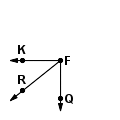mQFR = _________mRFK = 398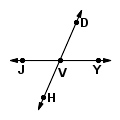mYVD = _________mJVH = 669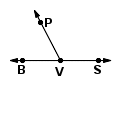mBVP = _________mSVP = 11710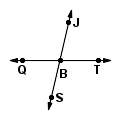mQBJ = _________mQBS = 7711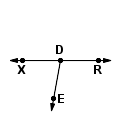mXDE = _________mRDE = 10012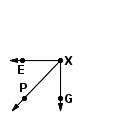mGXP = _________mPXE = 47